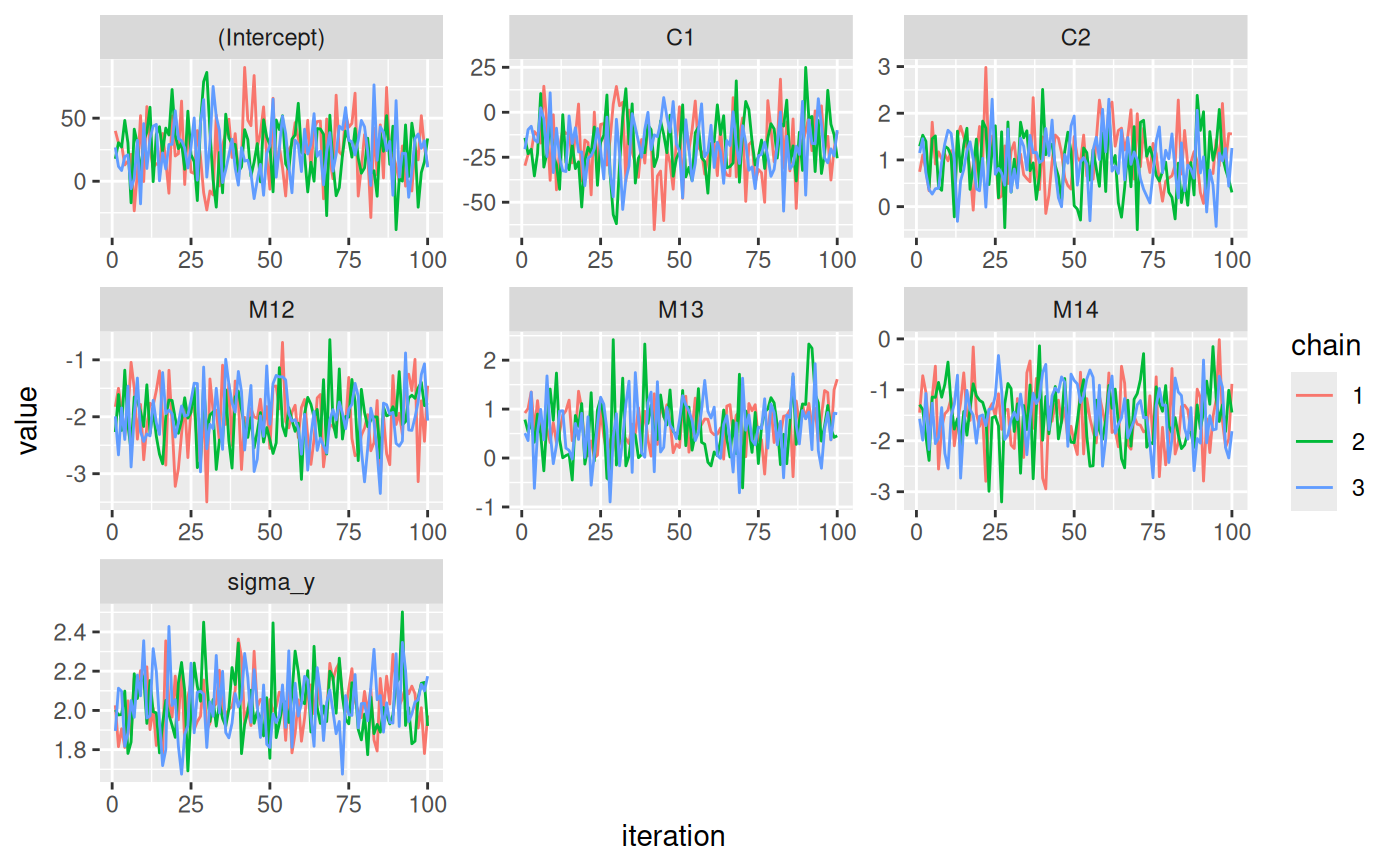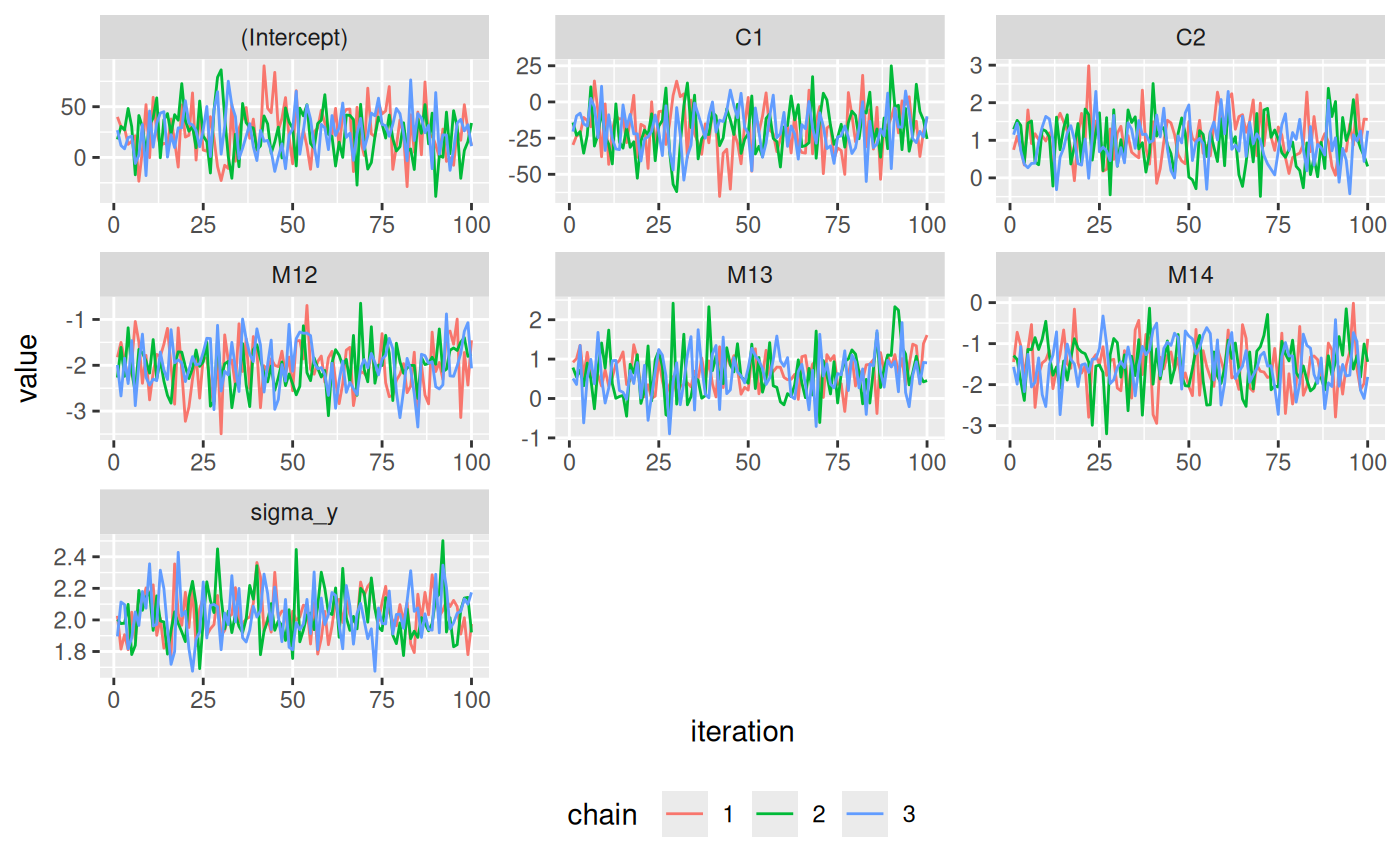Creates a set of traceplots from the MCMC sample of an object of class 'JointAI'.

traceplot(object, ...)

# S3 method for JointAI
traceplot(object, start = NULL, end = NULL,
thin = NULL, subset = c(analysis_main = TRUE), outcome = NULL,
exclude_chains = NULL, nrow = NULL, ncol = NULL, use_ggplot = FALSE,
warn = TRUE, mess = TRUE, ...)

## Arguments

object

object inheriting from class 'JointAI'

...

Arguments passed on to graphics::matplot

lty,lwd,lend

vector of line types, widths, and end styles. The first element is for the first column, the second element for the second column, etc., even if lines are not plotted for all columns. Line types will be used cyclically until all plots are drawn.

col

vector of colors. Colors are used cyclically.

cex

vector of character expansion sizes, used cyclically. This works as a multiple of par("cex"). NULL is equivalent to 1.0.

bg

vector of background (fill) colors for the open plot symbols given by pch = 21:25 as in points. The default NA corresponds to the one of the underlying function plot.xy.

add

logical. If TRUE, plots are added to current one, using points and lines.

verbose

logical. If TRUE, write one line of what is done.

start

the first iteration of interest (see window.mcmc)

end

the last iteration of interest (see window.mcmc)

thin

thinning interval (integer; see window.mcmc). For example, thin = 1 (default) will keep the MCMC samples from all iterations; thin = 5 would only keep every 5th iteration.

subset

subset of parameters/variables/nodes (columns in the MCMC sample). Follows the same principle as the argument monitor_params in *_imp.

outcome

optional; vector identifying a subset of sub-models included in the output, either by specifying their indices (using the order used in the list of model formulas), or their names (LHS of the respective model formula as character string)

exclude_chains

optional vector of the index numbers of chains that should be excluded

nrow

optional; number of rows in the plot layout; automatically chosen if unspecified

ncol

optional; number of columns in the plot layout; automatically chosen if unspecified

use_ggplot

logical; Should ggplot be used instead of the base graphics?

warn

logical; should warnings be given? Default is TRUE.

mess

logical; should messages be given? Default is TRUE.

summary.JointAI, *_imp, densplot
The vignette Parameter Selection contains some examples how to specify the parameter subset.

## Examples

# fit a JointAI model
mod <- lm_imp(y ~ C1 + C2 + M1, data = wideDF, n.iter = 100)

# Example 1: simple traceplot
traceplot(mod)# Example 2: ggplot version of traceplot
traceplot(mod, use_ggplot = TRUE)# Example 5: changing how the ggplot version looks (using ggplot syntax)
library(ggplot2)

traceplot(mod, use_ggplot = TRUE) +
theme(legend.position = 'bottom') +
xlab('iteration') +
ylab('value') +
scale_color_discrete(name = 'chain')